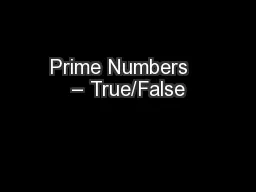# Prime Numbers – True/False2018-02-21 24K 24 0 0

## Prime Numbers – True/False - Description

There is a rule for finding any prime (. eg. the . ). . There . is a way to find out how many primes are below any number (. eg. Number of primes below 1000). . There . is no end to the prime numbers. . ID: 633633 Download Presentation

Embed code:

## Prime Numbers – True/False

Download Presentation - The PPT/PDF document "Prime Numbers – True/False" is the property of its rightful owner. Permission is granted to download and print the materials on this web site for personal, non-commercial use only, and to display it on your personal computer provided you do not modify the materials and that you retain all copyright notices contained in the materials. By downloading content from our website, you accept the terms of this agreement.

### Presentations text content in Prime Numbers – True/False

Slide1

Prime Numbers – True/False

There is a rule for finding any prime (eg the ). There is a way to find out how many primes are below any number (eg Number of primes below 1000). There is no end to the prime numbers. There is no end to primes only 2 apart (eg 17 & 19). All primes are one more or less than a multiple of 6. Any even number greater than 2 can be written as the sum of two primes (eg ). Every number greater than 1 can be written uniquely as the product of primes (eg ). \$100,000 was offered to factorise a 309 digit number.

Slide2

Prime Numbers – True/False

1. There is a rule for finding any prime (eg the ). (The prime is , but we worked it out the hard way)FALSE-ishWe know some about what it isn’t… produces primes for to The rounded down part of

is prime for all

, but the catch is that we don’t know what

is (and currently can only calculate it using primes, so it’s a bit of a circular formula)

Slide3

Prime Numbers – True/False

2. There is a way to find out how many primes are below any number (eg Number of primes below 1000). (There are primes below 1000, but we worked it out the hard way)True-ishMathematicians are working on it, and there are better and better ways of finding new primes, but so far there is no easy way to find the number of primes below . We do have a name for the formula: . And we know that

for

, so that’s a start…

Slide4

Prime Numbers – True/False

3. There is no end to the prime numbers. TrueWe can prove this by assuming there is a fixed number:Multiply all the primes together, then add 1. This number doesn’t divide by any of the primes, but all numbers greater than 1 either are prime or divide by primes. So this number either divides by primes we didn’t use, or is itself a prime we didn’t use. But we used them all!

Slide5

Prime Numbers – True/False

4. There is no end to primes only 2 apart (eg 17 & 19). (We’ve found the first 808,675,888,577,436 of them, but we did it the long way)UnknownThis idea is called the ‘twin primes conjecture’. Many mathematicians think it’s true (and even that there are an infinite number of primes 4 apart, 6 apart, 8 apart, etc) but we haven’t been able to prove it yet.

Slide6

Prime Numbers – True/False

5. All primes are one more or less than a multiple of 6.Nearly TrueIf a number is 3 away from a multiple of 6, it divides by 3. If a number is 2 or 4 away from a multiple of 6, it’s even. Therefore all primes apart from and must be 1 away.

Slide7

Prime Numbers – True/False

6. Any even number greater than 2 can be written as the sum of two primes (eg ). (It’s true for the first 4,000,000,000,000,000,000 numbers, but we worked it out the long way)UnknownKnown as Goldbach’s Conjecture, we suspect this to be true (no counter-examples have ever been found), but it is still unproven.

Slide8

Prime Numbers – True/False

7. Every number greater than 1 can be written uniquely as the product of primes (eg ). TrueThis fact is so important it has its own name - the Fundamental Theorem of Arithmetic (FTA)The proof follows from the basic properties of division, and follows from the idea that a factor of is a factor of either or .

Slide9

Prime Numbers – True/False

8. \$100,000 was offered to factorise a 309 digit number.TrueRSA encryption is based on the difficulty of factorising large numbers. Two large primes are multiplied together to produce a ‘key’ meaning anyone can encrypt data to send to you, but only you - the person who generated the key - can decrypt it. The only known way to break the code is to split the key into its prime factors.